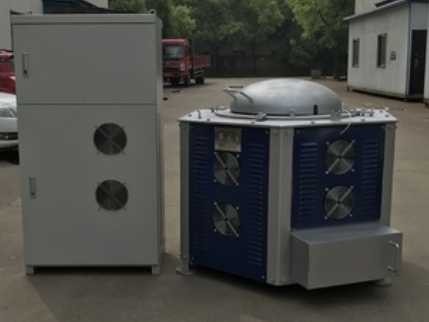### the energy loss summary of the induction heating process of the intermediate frequency aluminum melting furnace:

the energy loss summary of the induction heating process of the intermediate frequency aluminum melting furnace:

1. Heat loss due to current flowing through the inductor: This loss is carried away by the cooling water. This part of the loss is the main loss of the system, which is related to the structure of the inductor, the physical properties of the charge, and the heating frequency. The “electrical efficiency” is commonly used to describe this loss. Electrical efficiency is the ratio of the energy delivered to the heated workpiece to the energy obtained by the inductor from the power source.

2 , heat loss: the loss caused by the heat of the heated workpiece to the surrounding, this part of the loss is second only to the inductor loss. The commonly used “thermal efficiency” describes the loss, which is the ratio of the net heat that heats the workpiece to the total heat that the workpiece receives from the induction coil.

3. Transmission loss: The loss caused by the cable and busbar from the variable frequency power supply to the load is generally 2% to 7% .

4 , conversion loss: the converter components in the power cabinet, filter inductors, converter inductors, compensation capacitor losses, generally 2% ~ 5% .

6, intermediate frequency melting furnace efficiency of 62%, the thermal efficiency can reach 75% conversion loss is 3%, the transmission loss is 5%.the energy loss summary of the induction heating process of the intermediate frequency aluminum melting furnace:

1. Heat loss due to current flowing through the inductor: This loss is carried away by the cooling water. This part of the loss is the main loss of the system, which is related to the structure of the inductor, the physical properties of the charge, and the heating frequency. The “electrical efficiency” is commonly used to describe this loss. Electrical efficiency is the ratio of the energy delivered to the heated workpiece to the energy obtained by the inductor from the power source.

2 , heat loss: the loss caused by the heat of the heated workpiece to the surrounding, this part of the loss is second only to the inductor loss. The commonly used “thermal efficiency” describes the loss, which is the ratio of the net heat that heats the workpiece to the total heat that the workpiece receives from the induction coil.

3. Transmission loss: The loss caused by the cable and busbar from the variable frequency power supply to the load is generally 2% to 7% .

4 , conversion loss: the converter components in the power cabinet, filter inductors, converter inductors, compensation capacitor losses, generally 2% ~ 5% .

6, intermediate frequency melting furnace efficiency of 62%, the thermal efficiency can reach 75% conversion loss is 3%, the transmission loss is 5%.